#### You may also like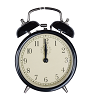### On Time

On a clock the three hands - the second, minute and hour hands - are on the same axis. How often in a 24 hour day will the second hand be parallel to either of the two other hands?### Estimating Angles

How good are you at estimating angles?### LOGO Challenge 1 - Star Square

Can you use LOGO to create this star pattern made from squares. Only basic LOGO knowledge needed.

# Shogi Shapes

##### Age 11 to 14Challenge Level

Arnav from Franconian International School in Germany began by thinking about angles in a nonagon from the Getting Started section:

What angle do you turn through altogether with a nonagon?
Answer: 360 because 360 degrees is a complete circular turn and a nonagon lands on the same dot you started with every time you turn 360 degrees.
What must each exterior angle (the red angles) be?
Answer: 40 because 360$\div$9 = 40
What must each interior angle be?
Answer: 140 because 180$-$40 = 140

Luxsija from Mill Hill County High School, Year 7 students from Chesterfield High School, Oliver from The Priory Academy LSST and Rishika from Nonsuch High School for Girls, all in the UK, and Ashlynn from ISF Academy in Hong Kong found the angles in the octagon and kites. This is Oliver's work: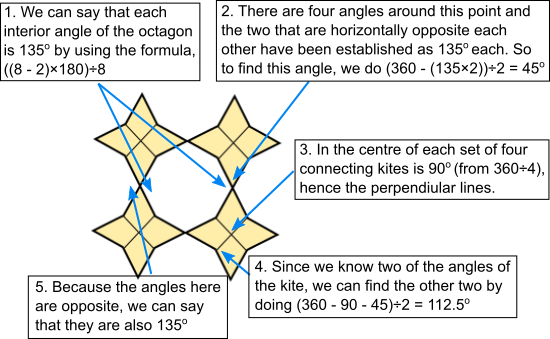For the decagon and the rhombuses, Rishika and the Chesterfield year 7 students started with the decagon. This is Luxsija's work: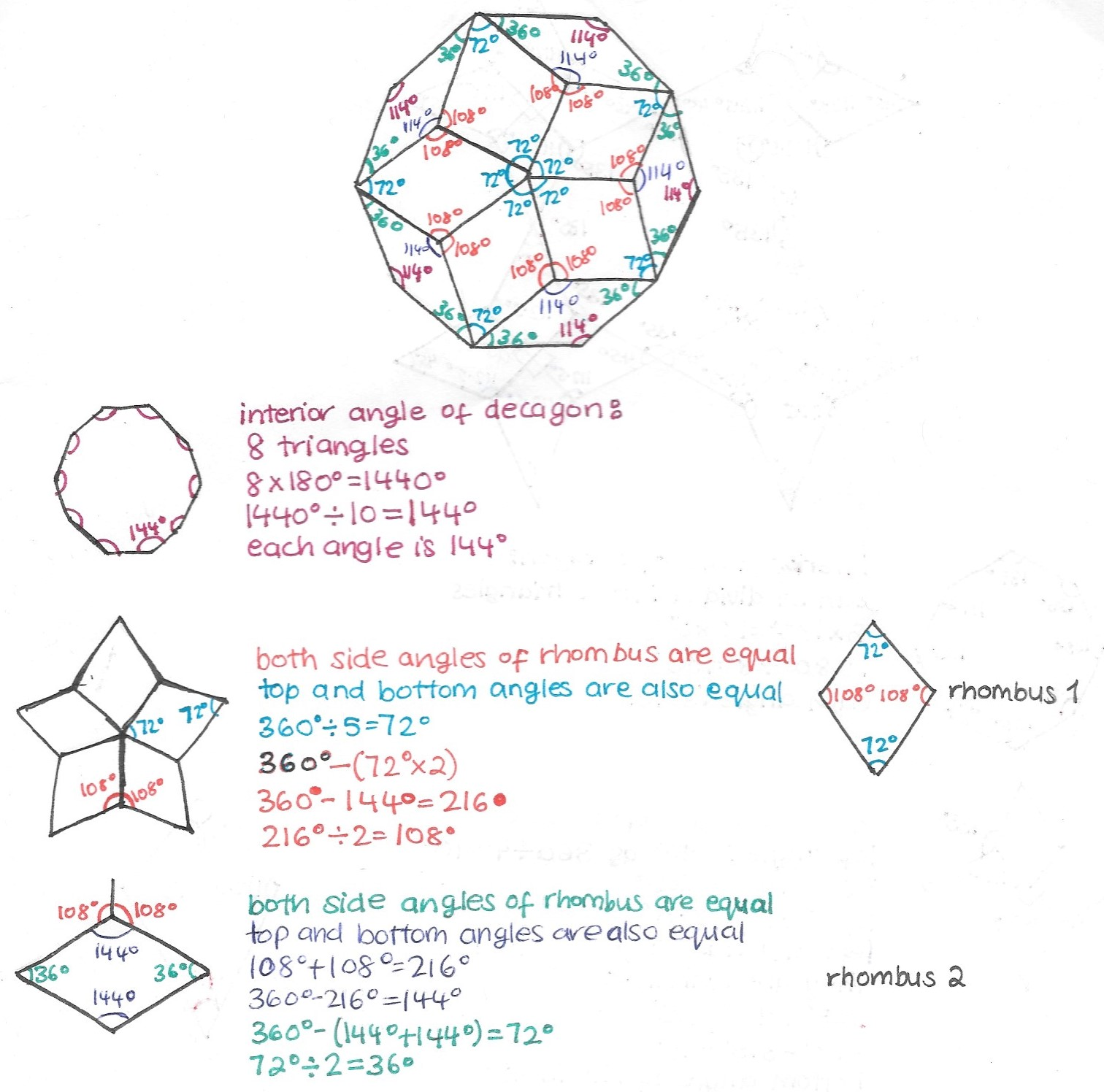Ashlynn and the Chesterfield year 7s started with the rhombuses. This is the Chesterfield year 7s' work: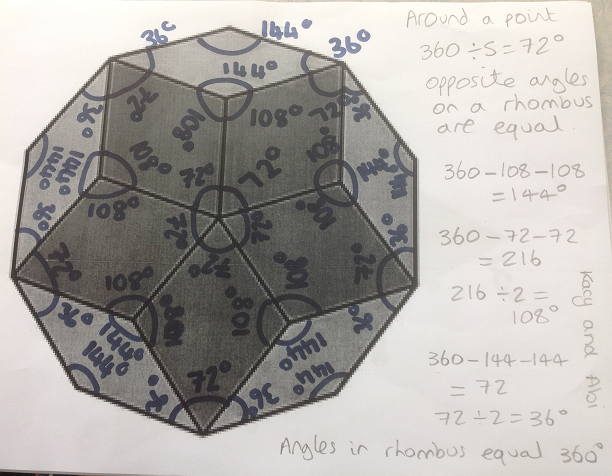They used the facts that the angles at a point (in the centre) add up to 360$^\circ$, opposite angles in a rhombus are equal, and angles in a rhombus add up to 360$^\circ$.

For the triangle and the nonagon, Rishika, Luxsija, Ashlynn and Chesterfield year 7 all began by finding the interior angles of each shape. This is Rishika's work: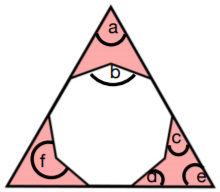As it is an equilateral triangle, angles are equal. Angles in a triangle add to $180^\circ$, and therefore, angle $\text{a}=180\div3 = 60^\circ.$

Sum of interior angles in a nonagon is:
$(n-2)\times180 = (9-2)\times180 = 1260$

Therefore, angle $\text{b}$ is $1260\div9 = 140^\circ$

Angle $\text{c}$ and angle $\text{d}$, which are equal, are $180^\circ-\text{angle b}$ (because they are on a straight line), so $\text{angle b}= 180-140 = 40^\circ$

As angles in a quadrilateral add to $360^\circ$, $\text{angle f}=360 - \text c-\text d-\text a = 360 - 40-40-60 = 220^\circ$
This is verified as $\text f+\text b$ should equal $360^\circ$ as it is around a point ($140+220 = 360,$ so it is correct).

For the other nonagon, Luxsija and Chesterfield year 7s used kites and parallelograms inside the shapes. This is Luxsija's work. The pink shapes are not actually parallelograms, but they are quadrilaterals so the angles still add up to 360$^\circ$: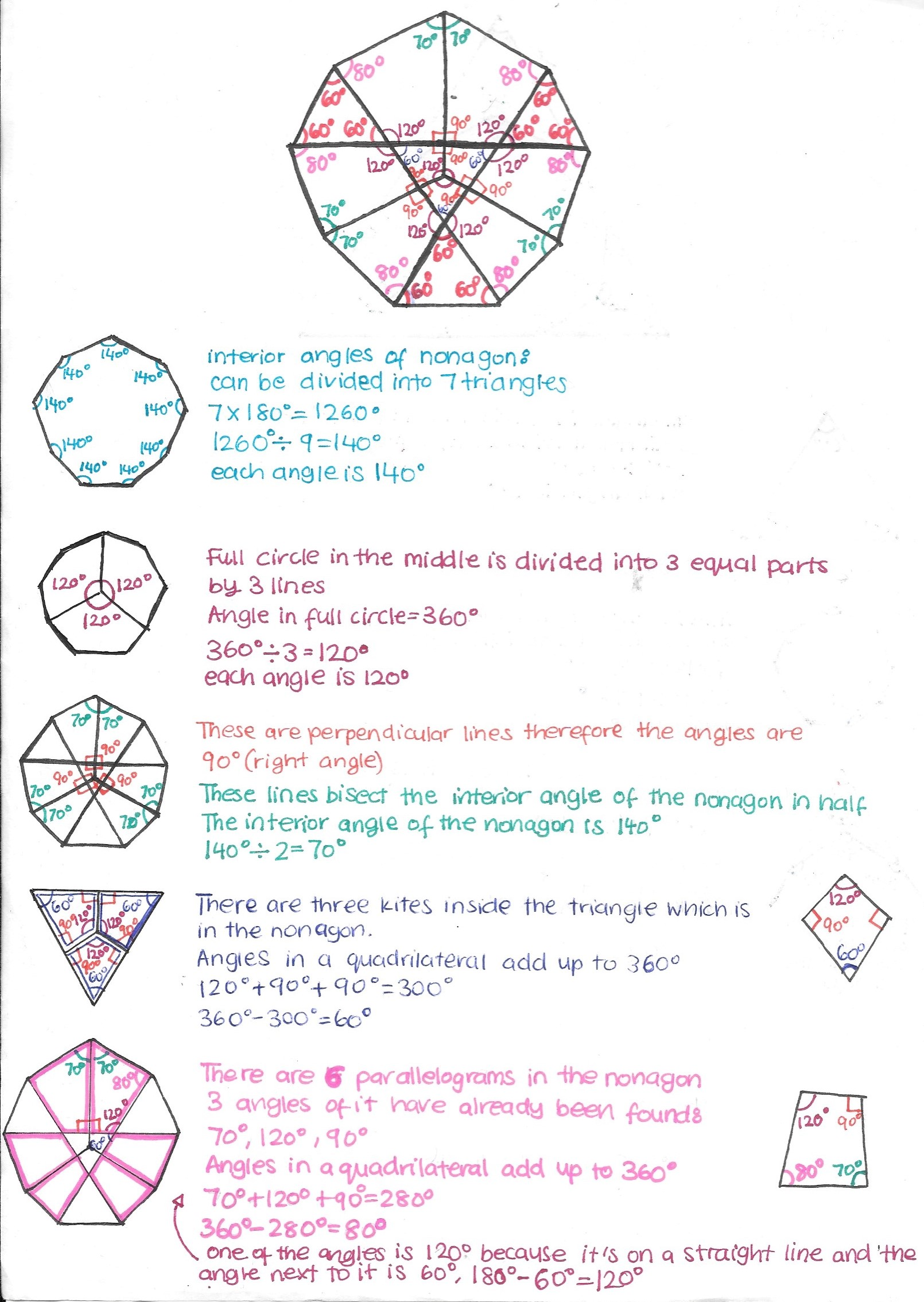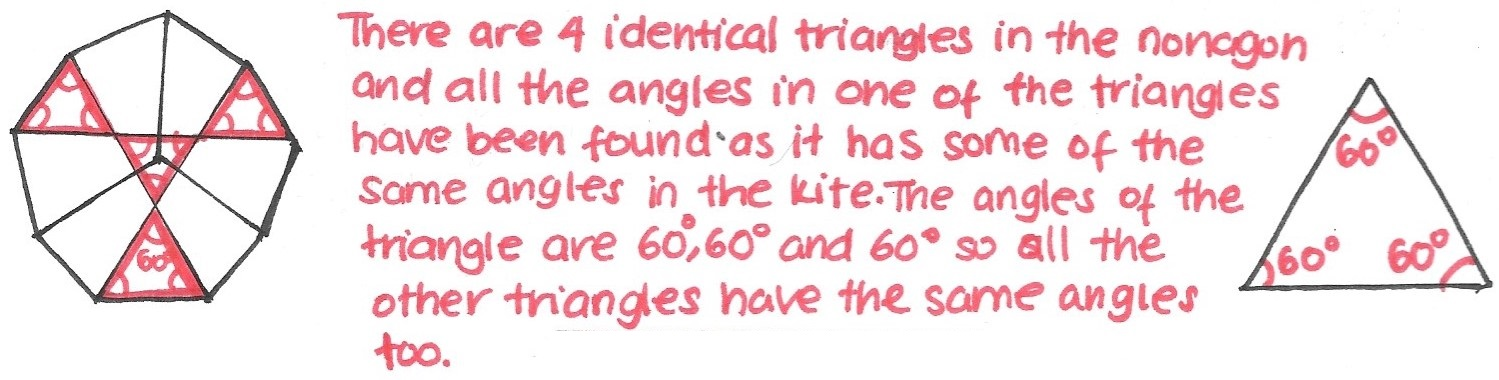Rishika used symmetry and properties of angles in triangles and on lines and points: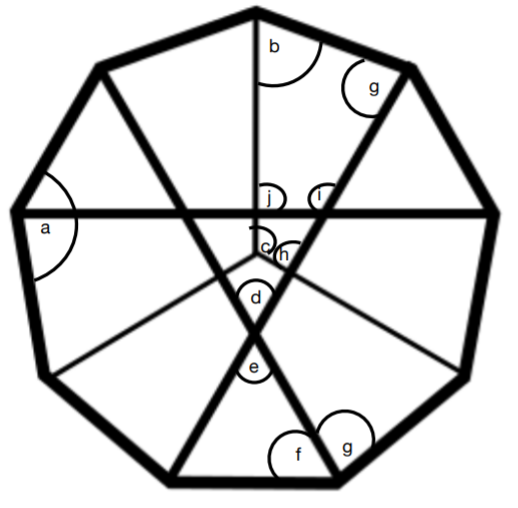Angle $\text{a}$ is established to be $140^\circ$ (see above).

Angle $\text{b}$ is half of angle $\text{a}$, which is $0.5\times140 = 70^\circ$

Angle $\text{c}$ is $360\div3$ (as 3 fit around a point) $= 120^\circ$

Angle $\text{d}$ is an angle in an equilateral triangle, and so must be $60^\circ$ as all angles are equal and angles in a triangle add to $180^\circ$

Angle $\text{e}$ is equal as it is vertically opposite, and therefore is $60^\circ$ as well.

Angle $\text{f}$ is part of an equilateral triangle $= 60^\circ$.

Angle $\text{g} =\text a - \text f$ as $\text{g} = 140 - 60 = 80^\circ$

Angle $\text{h}$ must be $360 - \text c - \text b - \text g$ as angles in a quadrilateral add to
$360^\circ$.
$360 -70-120 -80 = 90^\circ$

Also, angles $\text{g , d}$ and $\text{a}$ can be verified as they form a kite and must add to $360^\circ$. $80+80+140+60 = 360^\circ$ and therefore, they are correct.

Angle $\text{i}$ must be $(360 - 60-60)\div2$ as angles around a point add to $360^\circ$.
Angle $\text{i} = 120^\circ$.

Angle $\text{j}$ must be $360^\circ - 70 - 80 - 120 = 90^\circ$

Ashlynn used a pentagon: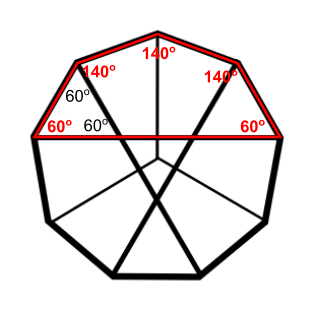The exterior angle of nonagon is $360\div9=40^\circ$

The interior angle of nonagon is $180-40=140^\circ$

In the red pentagon, there are three $140^\circ$ angles. The small angle is equal to $(540^\circ-3\times140^\circ)\div2=60^\circ$

All the small triangles have angles of $60^\circ$ and are equilateral.

Luxsija, Rishika and Ashlynn all counted the shogi tiles and found some angles. This is Rishika's work: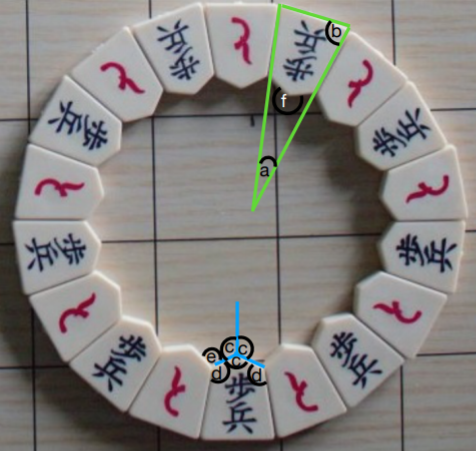The tiles are in pairs - there are $9$ pairs. $2\times9 = 18.$

The tiles are congruent, irregular pentagons.

The shape inside is $36$-sided (each tile provides $2$ sides), so the sum of the interior angles is:
$(36-2)\times180^\circ=6120^\circ$

However, we have to remember that this includes $18$ angles [smaller than $90^\circ$] and $18$ [reflex] angles.

We can work out angle $\text a$ because the shogi pieces are arranged to make a circle - $360^\circ$. There are $18$ tiles, so $18\text a=360^\circ\Rightarrow\text a = 20^\circ$

As the triangle formed is isosceles, we can work out angle $\text b$ as angles in a triangle add to $180^\circ.$
$\text b=(180-20)\div2=80^\circ$

$3\times\text{angle c}$ fits around a point, and therefore it must be $120^\circ.$

From there, Rishika found angle $\text d$ from the sum of the interior angles in a pentagon, and angle $\text e$ from the sum of angles at a point. However, angle $\text c$ is not neccessarily $120^\circ.$ This is Ashlynn's work on the angles in the tiles:
If we assume that the tiles are symmetrical, there are two pairs of matching angles and one other angle. What is the range of possible sizes of that "lonely angle" (angle $\text c$)?

The smallest angle is when it becomes a triangle i.e. $20^\circ$ (so angle $\text c$ is at angle $\text a$)
The biggest angle is when it becomes a trapezoid i.e. $180^\circ$